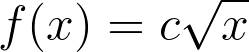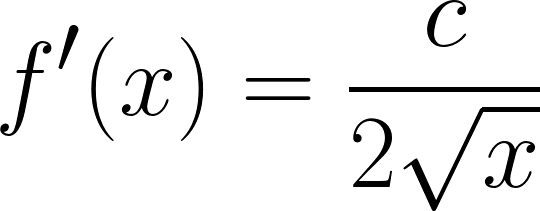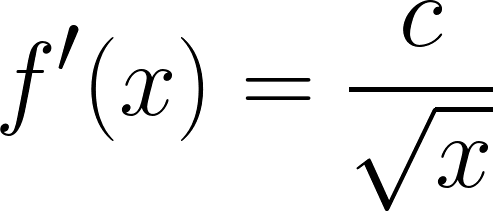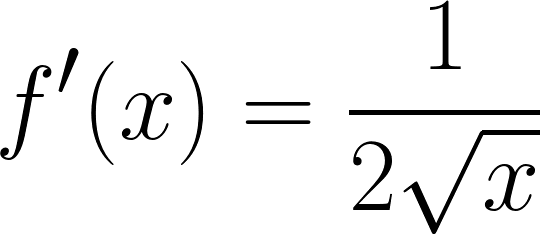### Day 17 - Derivative Rules - 01.27.16

 Update Unit 2 TestFriday, 02.12.16 What is the derivative of the following: f(x) = cf’(x) = cf’(x) = 1f’(x) = 0does not existnone of the aboveWhat is the derivative of the following: f(x) = cxf’(x) = cf’(x) = 1f’(x) = 0does not existnone of the aboveWhat is the derivative of the following: f(x) = cx2f’(x) = 2cxf’(x) = 2cf’(x) = cf’(x) = 0none of the aboveWhat is the derivative of the following:does not existnone of the aboveWhat is the derivative of the following: f(x) = x2 + x + 1f’(x) = 2xf’(x) = 2x + 1f’(x) = x + 1does not existnone of the aboveReviewPre-calculusSlopeEquation of a LineSecant Line vs. Tangent Line (video)DerivativeHow can the slope of a tangent line be found? Equation of a Tangent Line (video)Tangent Line GraphDerivative (video)LessonBasic Differentiation RulesConstant Rule (video) (example)Power Rule (video) (example)Constant Multiple Rule (video) (example)Sum and Difference Rule (video)Derivative of Sine and Cosine FunctionsCheckpoints Exit Ticket Posted on the board at the end of the blockHomeworkWNQ the following:Product Rule (video)Quotient Rule (video)Study! Help Request List Standard(s) APC.2Define and apply the properties of limits of functions.Limits will be evaluated graphically and algebraically.Includes:​limits of a constant​limits of a sum, product, and quotient​one-sided limits​limits at infinity, infinite limits, and non-existent limitsAPC.3Use limits to define continuity and determine where a function is continuous or discontinuous.Includes:​continuity in terms of limitscontinuity at a point and over a closed interval​application of the Intermediate Value Theorem and the Extreme Value Theorem​geometric understanding and interpretation of continuity and discontinuity